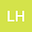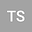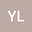Revisiting daily MODIS evapotranspiration algorithm using flux tower measurements in China
•••• +5Ronglin Tang
Institute of Geographical Science and Natural Resources Research
Author ProfileJunqing Zheng
Tsinghua University
Author ProfileChen Qiao
Tsinghua University
Author Profile## Abstract

Evapotranspiration (ET) is the major component of the hydrology cycle. Satellites provide a convenient way for gathering information to estimate regional ET. The most widely applied method for converting the instantaneous satellite measurement to daily scale assumes that evaporative fraction (EF), defined as the ratio of ET to the available energy, is constant during the daytime. However, this method was proved to underestimate the daily ET. This study implemented a theoretically improved EF algorithm to calculate daily ET with the decoupling factor method based on the Penman-Monteith and McNaughton-Jarvis equations. Seven improved algorithms were developed by assuming that various parameters remain constant during the day. The satellite-based ET estimates were compared with seven local flux tower measurements in China. The results showed that: (1) The original ET method calculated the daily evaporation more accurately than the other algorithms. However, the good fit was based on two compensating inaccuracies. Compared to the flux tower measurement, the original ET method underestimated the daily EF by 26% and overestimated the daily net radiation by 30%. (2) Six of the seven proposed algorithms underpredicted the daily ET by 30-60%, mainly due to the inaccurate daily net radiation. (3) The algorithm that assumed that the instantaneous decoupling parameter Ω* was equal to its daily value method calculated EF and ET with the relative errors of 8% and 10% when the inaccurate estimated daily net radiation was replaced by the observed flux tower data.